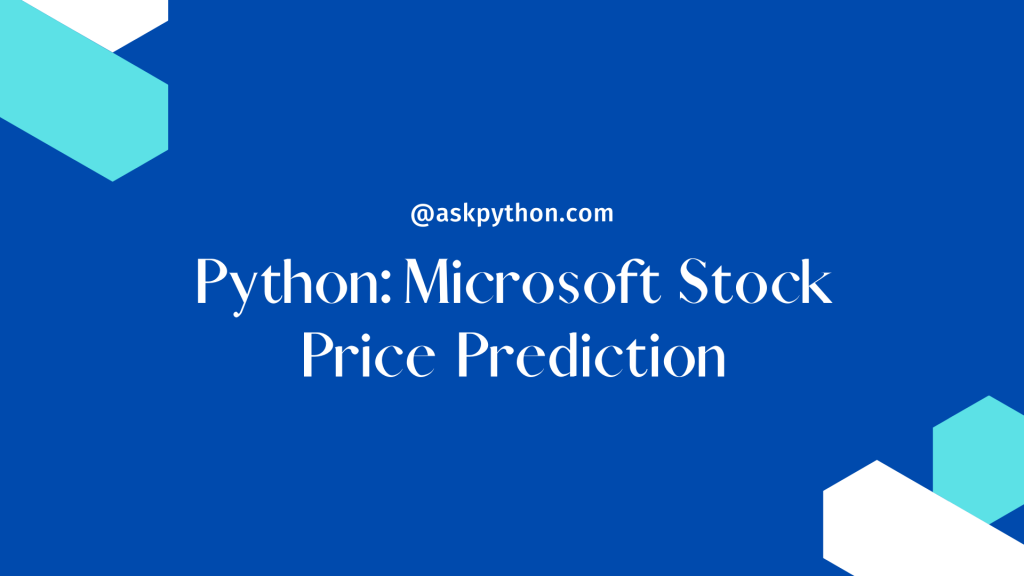# Microsoft Stock Price Prediction using PythonLet’s talk about Microsoft stock price prediction in this Python tutorial. Microsoft is now one of the world’s top technological corporations, employing over 163,000 people globally. It is well-known for producing the Windows operating system, which is one of the most widely used computer operating systems.

This post will teach you how to forecast Microsoft stock values in the future. In this essay, I will guide you through the process of predicting Microsoft stock prices with machine learning using Python.

## Importing necessary modules/libraries and the dataset

Let’s get started on the challenge of forecasting Microsoft stock prices by loading the relevant Python modules and dataset:

```import numpy as np
import pandas as pd
import matplotlib.pyplot as plt
import seaborn as sns
sns.set()
plt.style.use('seaborn')

```

## Data Visualization

The Close column in this dataset comprises the values whose future values we wish to anticipate. So, let’s take a deeper look at Microsoft’s stock price’s historical close prices:

```plt.figure(figsize=(10, 4))
plt.title("Microsoft Stock Prices")
plt.xlabel("Date")
plt.ylabel("Close")
plt.plot(data["Close"])
plt.show()
```

## Finding Co-relation between data

Let’s have a look at the correlation between the dataset’s characteristics now:

```print(data.corr())
sns.heatmap(data.corr(),cmap="Greens")
plt.show()
```

## Splitting Data into train and test data

I’ll now prepare the data for the machine learning model. In this phase, I’ll add the most significant characteristics to x and the target column to y, then divide the dataset into training and test sets:

```x = data[["Open", "High", "Low"]]
y = data["Close"]
x = x.to_numpy()
y = y.to_numpy()
y = y.reshape(-1, 1)

from sklearn.model_selection import train_test_split
xtrain, xtest, ytrain, ytest = train_test_split(x, y, test_size=0.2, random_state=42)
```

## Applying the machine learning model

Let’s now use the Decision Tree Regression Algorithm to train the Microsoft Stock Price prediction model and look at the projected stock prices for the next 5 days:

```from sklearn.tree import DecisionTreeRegressor
model = DecisionTreeRegressor()
model.fit(xtrain, ytrain)
ypred = model.predict(xtest)
data = pd.DataFrame(data={"Predicted Rate": ypred})
```